Ecological Archives E093-233-A1

Nathan P. Lemoine, Deron E. Burkepile. 2012. Temperature-induced mismatches between consumption and metabolism reduce consumer fitness. Ecology 93:2483–2489. http://dx.doi.org/10.1890/12-0375.1

Appendix A. Parameter and AIC estimates for all analyzed models and all supplementary figures.

TABLE A1. Parameter estimates, information criteria values, and posterior probabilities for each model evaluated for respiration rates, consumption rates, assimilation efficiency, and ingestion efficiency. k is Boltzmann’s constant, T is temperature (˚C for Null, Linear, and Brière1 models, K for exponential and Gaussian models), M is urchin mass. The model in bold represents the model with the best fit for each measurement. The inclusion of a mass term in the model means that the 95% confidence interval for the mass scaling parameter did not include 0.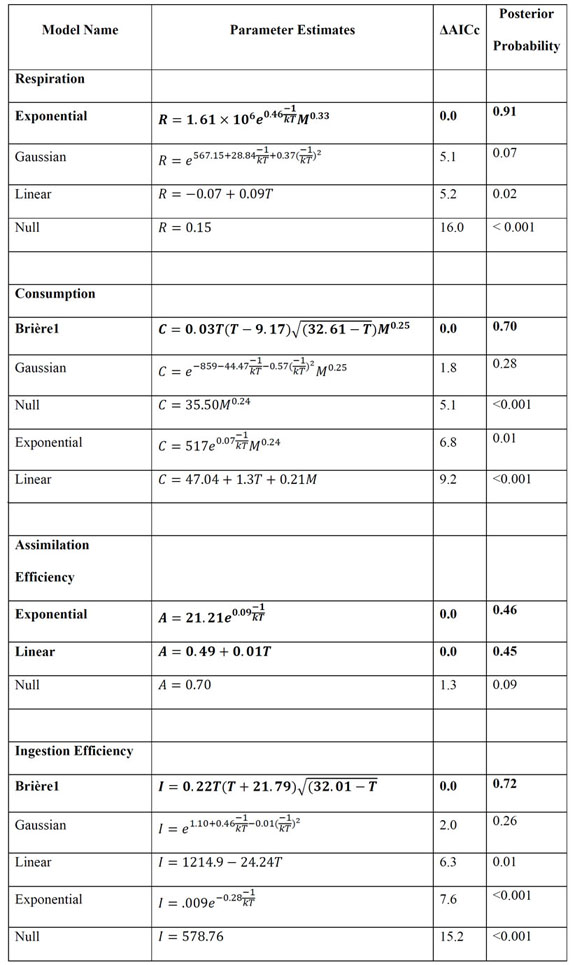TABLE A2. ΔAIC values for null, linear, and quadratic mixed effects models used in the meta-analysis.

 Model ΔAIC M:C ~ Temperature Linear 0.0 Quadratic 7.5 Null 15.6 Growth ~ M:C Quadratic 0.0 Linear 0.0 Null 5.8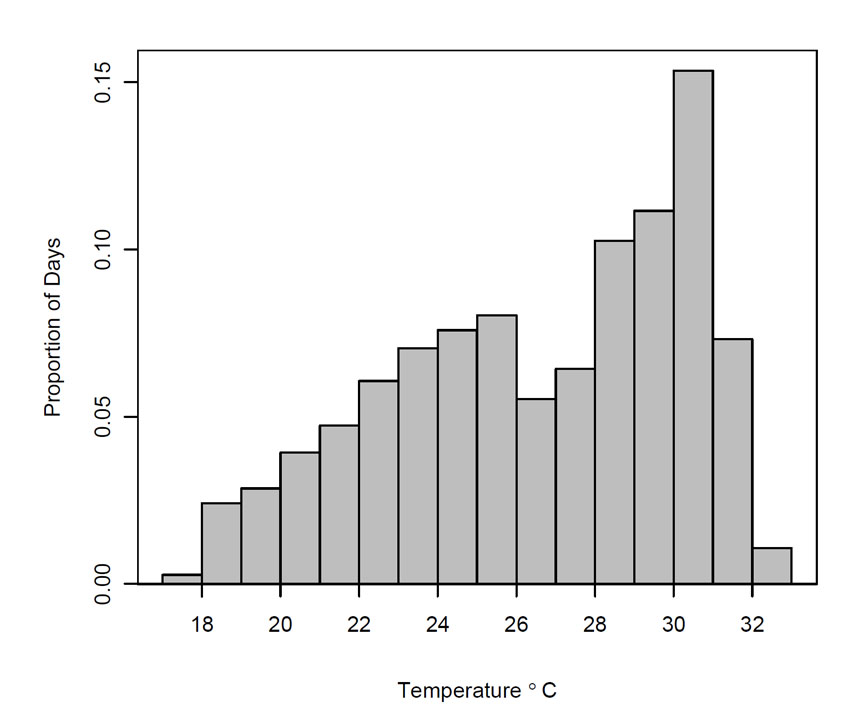FIG. A1. Histogram of average daily temperatures at Virginia Key from 2005–2010.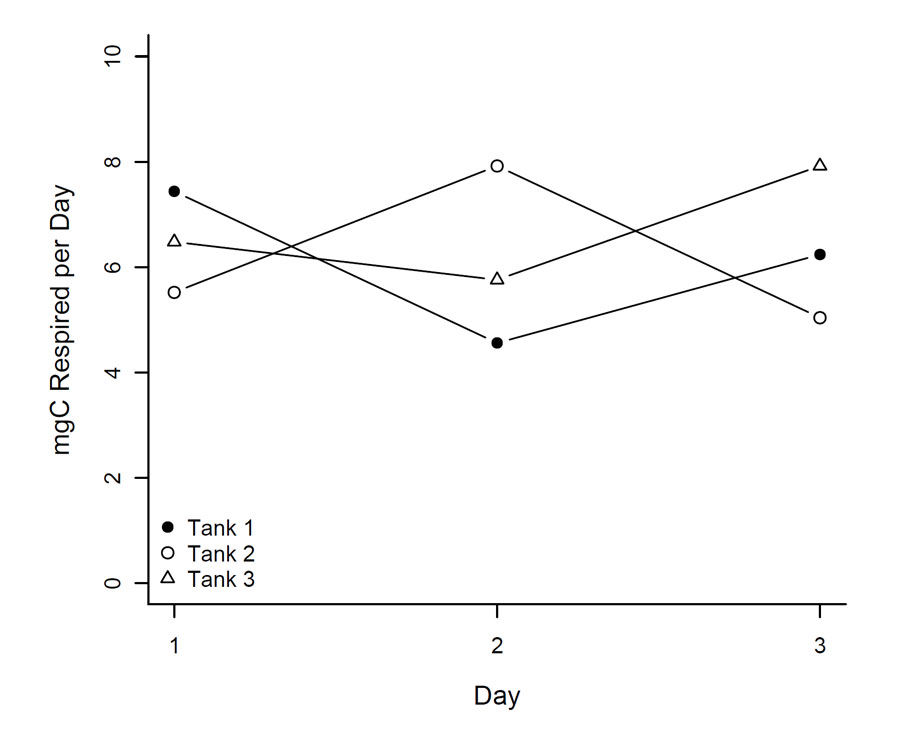FIG. A2. Metabolism of urchins held at 20˚C for three days.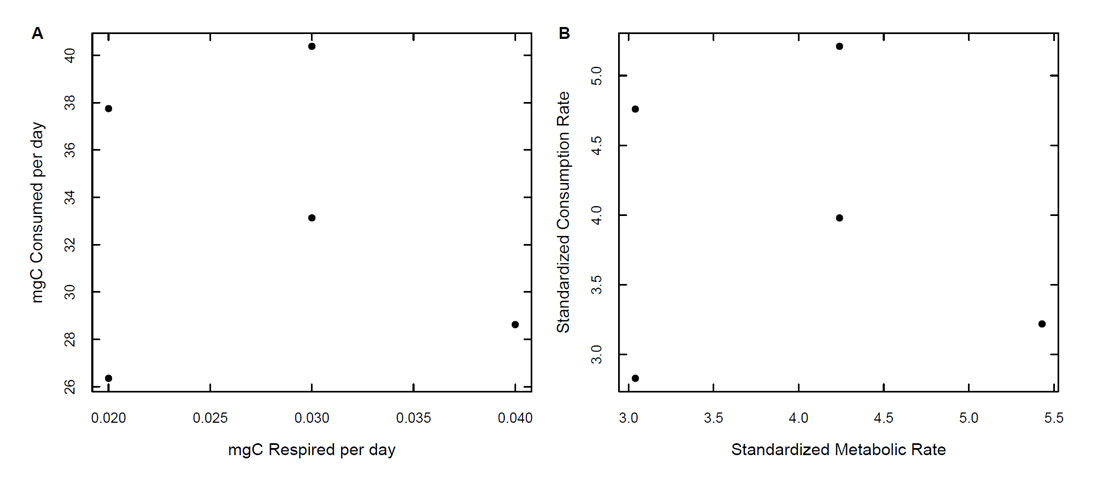FIG. A3. Comparison of the relationship between metabolism and consumption of L. variegatus on (A) the original measurement scale and (B) the standardized scale used in the meta-analysis. Note that the relationship remained constant after transformation.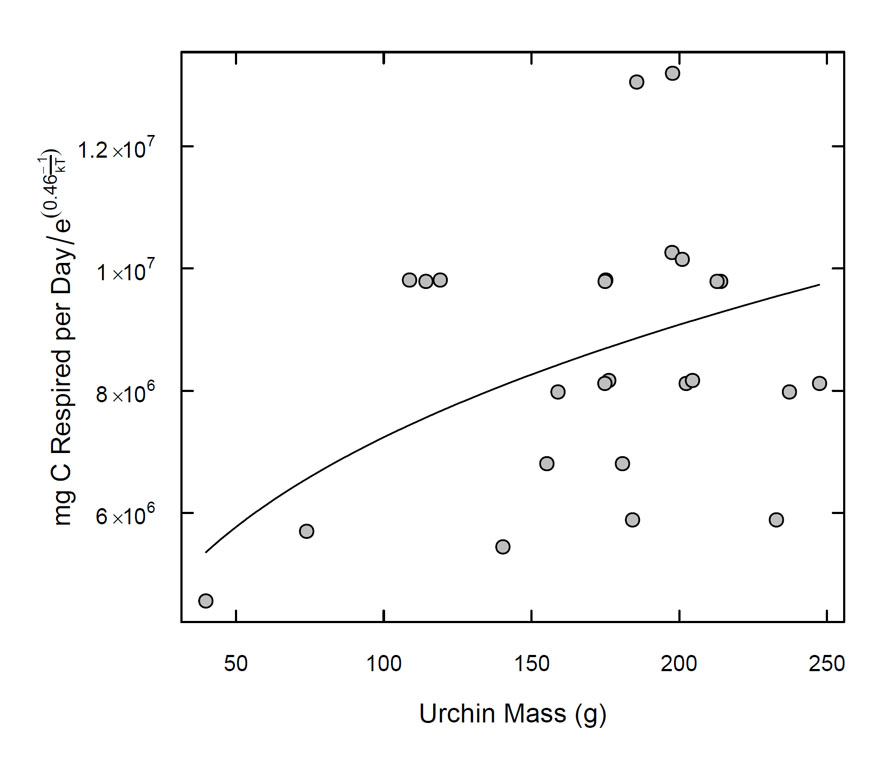FIG. A4. Relationship between temperature-corrected respiration rates and body mass for L. variegatus.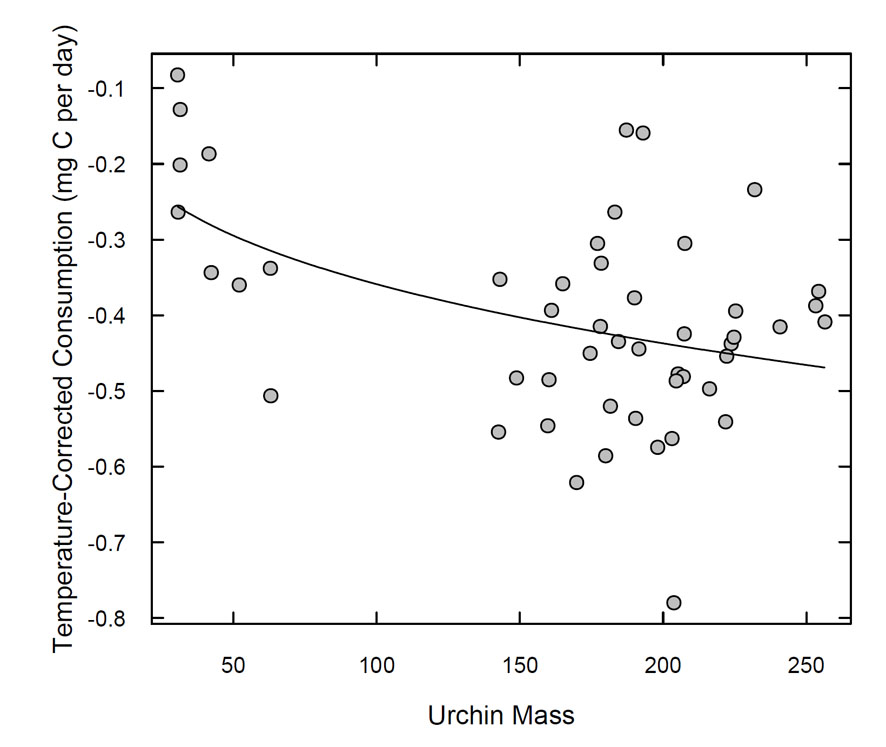FIG. A5. Relationship between temperature-corrected consumption rates and body mass for L. variegatus. Consumption rates were temperature corrected by dividing consumption by the temperature term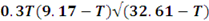(Table A1). Note that, due to the temperature correction factor, lower values indicate higher consumption rates. For example, at 26˚C, a temperature-corrected consumption value of -0.8 corresponds to an actual consumption of 270 mg C, whereas a temperature-corrected consumption value of -0.1 corresponds to an actual consumption of 34 mg C. Thus, the decline in temperature-corrected consumption indicates an increase in actual consumption rates with increasing body mass.